# RD Sharma Solutions for Class 9 Maths Chapter 10 Congruent Triangles Exercise 10.2

RD Sharma class 9 Mathematics chapter 10 exercise 10.2 Congruent Triangles solutions are provided here. The RD Sharma solutions have been systematically prepared for class 9 students to help them tackle all chapter 10 Maths problems. The solutions consists of solved exercises along with detailed explanations which have been given by BYJU’S experts. Students can easily access solved RD Sharma solutions for class 9 from the links given below to have an overview of the chapter.

## Download PDF of RD Sharma Solutions for Class 9 Maths Chapter 10 Congruent Triangles Exercise 10.2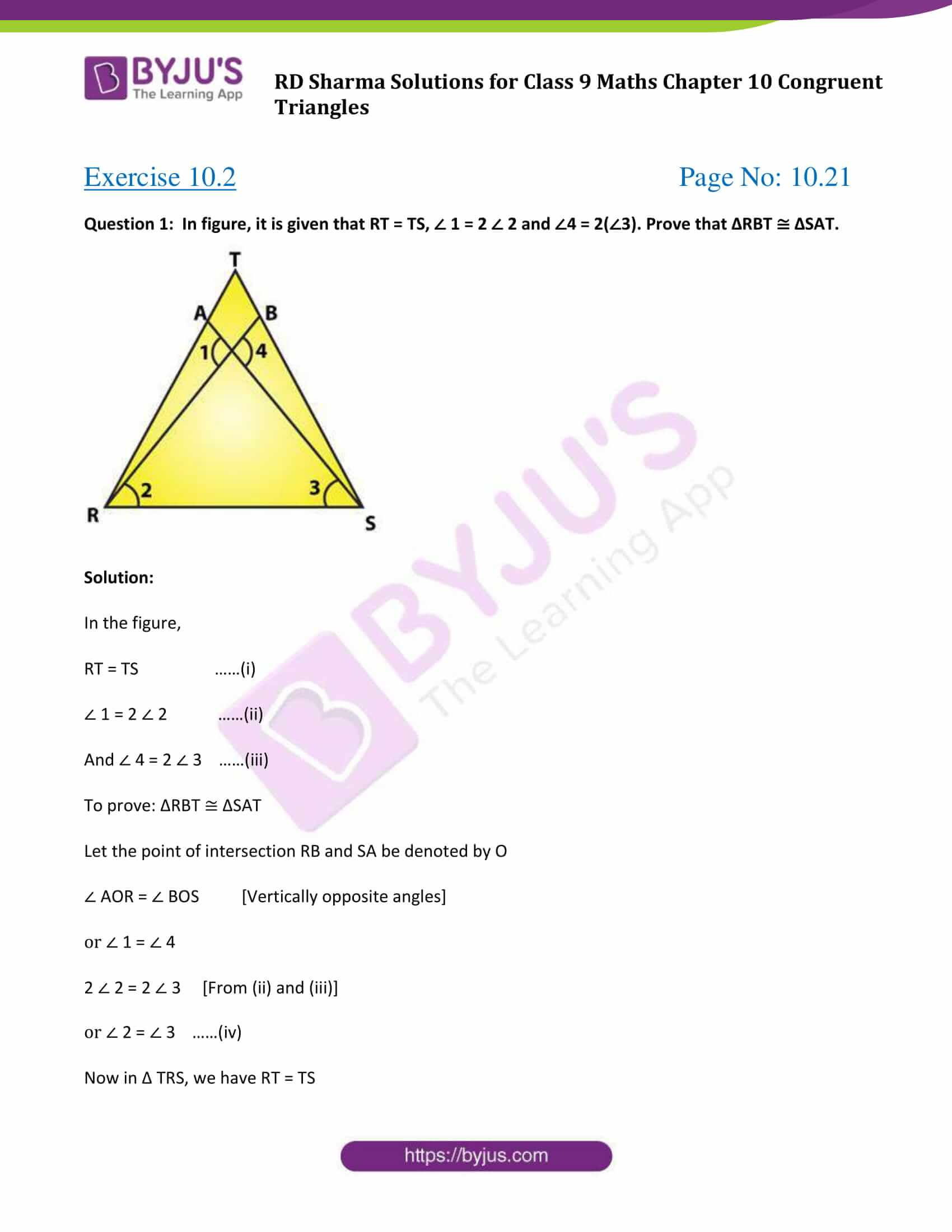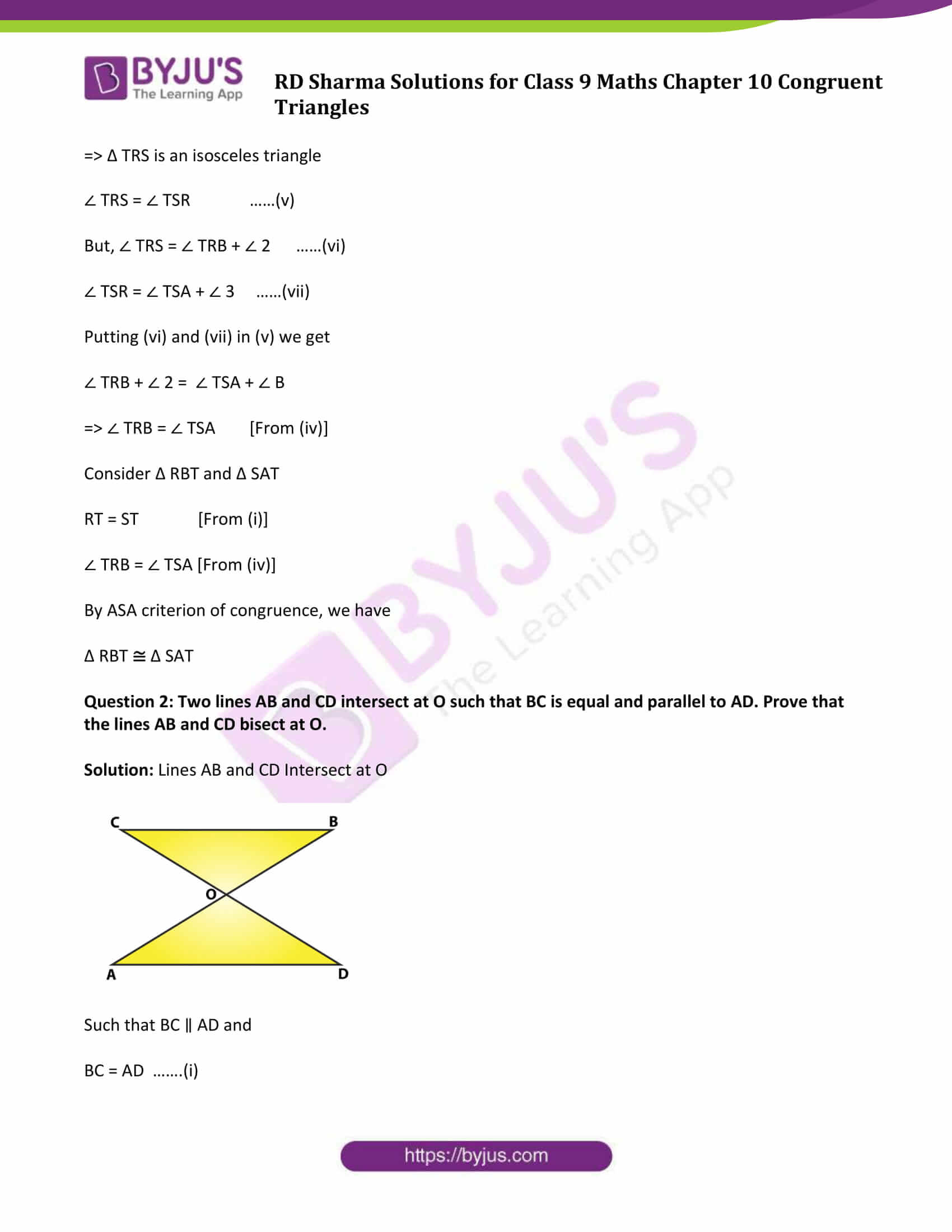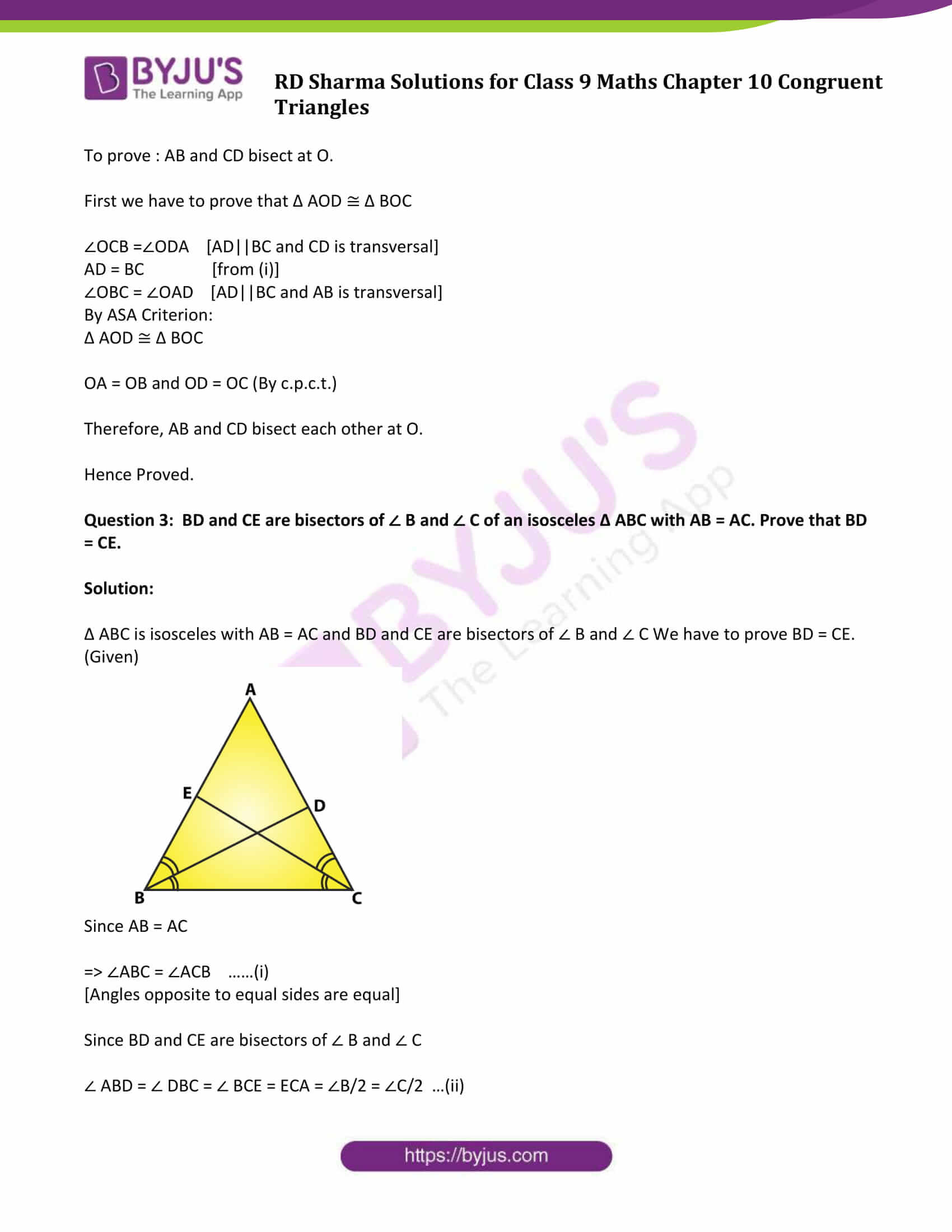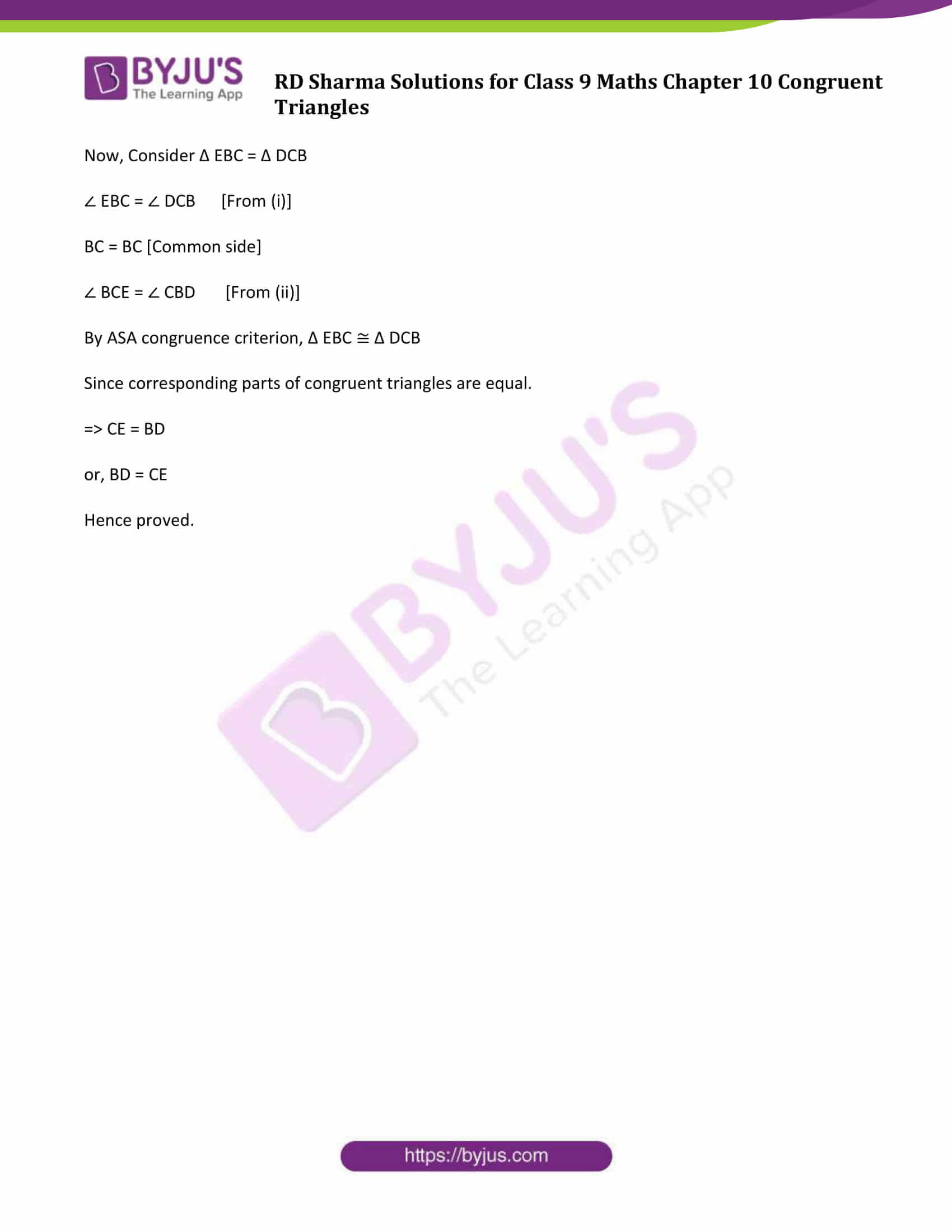### Access Answers to Maths RD Sharma Class 9 Chapter 10 Congruent Triangles Exercise 10.2 Page number 10.21

Question 1: In figure, it is given that RT = TS, ∠ 1 = 2 ∠ 2 and ∠4 = 2(∠3). Prove that ΔRBT ≅ ΔSAT.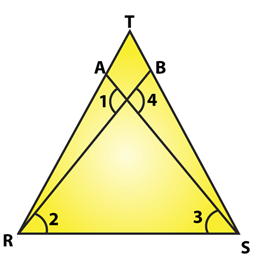Solution:

In the figure,

RT = TS ……(i)

∠ 1 = 2 ∠ 2 ……(ii)

And ∠ 4 = 2 ∠ 3 ……(iii)

To prove: ΔRBT ≅ ΔSAT

Let the point of intersection RB and SA be denoted by O

∠ AOR = ∠ BOS [Vertically opposite angles]

or ∠ 1 = ∠ 4

2 ∠ 2 = 2 ∠ 3 [From (ii) and (iii)]

or ∠ 2 = ∠ 3 ……(iv)

Now in Δ TRS, we have RT = TS

⇒ Δ TRS is an isosceles triangle

∠ TRS = ∠ TSR ……(v)

But, ∠ TRS = ∠ TRB + ∠ 2 ……(vi)

∠ TSR = ∠ TSA + ∠ 3 ……(vii)

Putting (vi) and (vii) in (v) we get

∠ TRB + ∠ 2 = ∠ TSA + ∠ B

⇒ ∠ TRB = ∠ TSA [From (iv)]

Consider Δ RBT and Δ SAT

RT = ST [From (i)]

∠ TRB = ∠ TSA [From (iv)]

By ASA criterion of congruence, we have

Δ RBT Δ SAT

Question 2: Two lines AB and CD intersect at O such that BC is equal and parallel to AD. Prove that the lines AB and CD bisect at O.

Solution: Lines AB and CD Intersect at O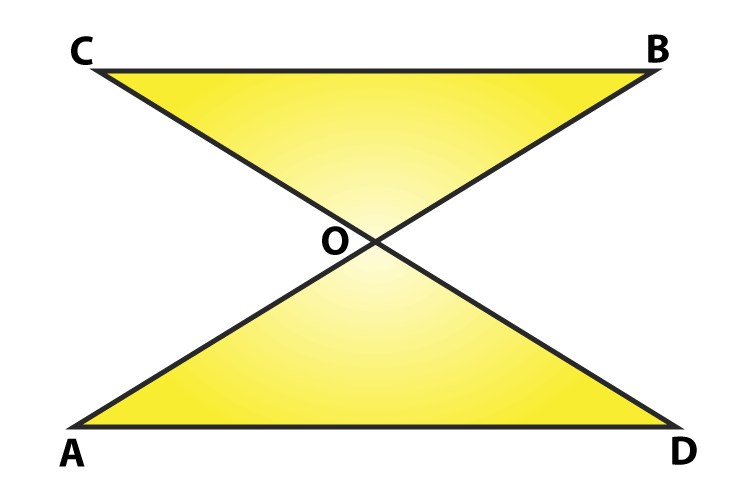Such that BC ∥ AD and

To prove : AB and CD bisect at O.

First we have to prove that Δ AOD ≅ Δ BOC

∠OCB =∠ODA [AD||BC and CD is transversal]

By ASA Criterion:

Δ AOD ≅ Δ BOC

OA = OB and OD = OC (By c.p.c.t.)

Therefore, AB and CD bisect each other at O.

Hence Proved.

Question 3: BD and CE are bisectors of ∠ B and ∠ C of an isosceles Δ ABC with AB = AC. Prove that BD = CE.

Solution:

Δ ABC is isosceles with AB = AC and BD and CE are bisectors of ∠ B and ∠ C We have to prove BD = CE. (Given)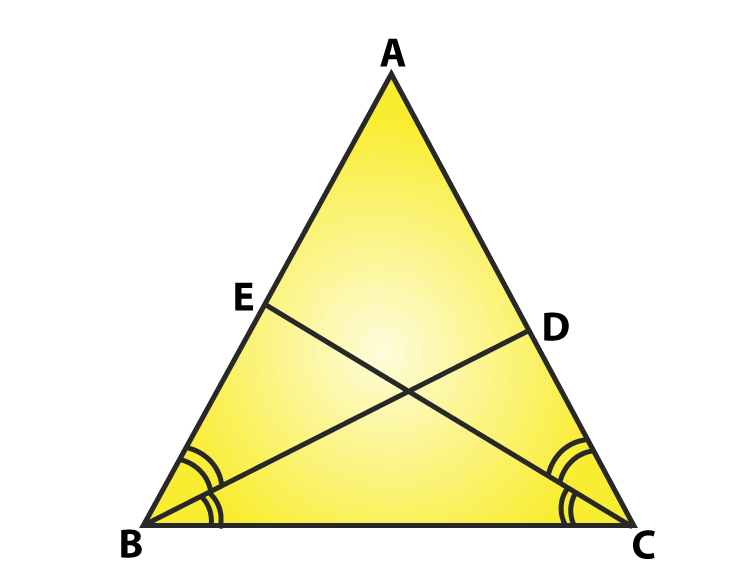Since AB = AC

⇒ ∠ABC = ∠ACB ……(i)

[Angles opposite to equal sides are equal]

Since BD and CE are bisectors of ∠ B and ∠ C

∠ ABD = ∠ DBC = ∠ BCE = ECA = ∠B/2 = ∠C/2 …(ii)

Now, Consider Δ EBC = Δ DCB

∠ EBC = ∠ DCB [From (i)]

BC = BC [Common side]

∠ BCE = ∠ CBD [From (ii)]

By ASA congruence criterion, Δ EBC ≅ Δ DCB

Since corresponding parts of congruent triangles are equal.

⇒ CE = BD

or, BD = CE

Hence proved.

## RD Sharma Solutions for Class 9 Maths Chapter 10 Exercise 10.2

RD Sharma Solutions Class 9 Maths Chapter 10 Congruent Triangles Exercise 10.2 is based on the topic- Criterion of Congruence – ASA ( Angle-Side-Angle). According to the criteria, two triangles are congruent if two angles and the included side of one triangle are equal to the corresponding two angles and included side of the other triangle.